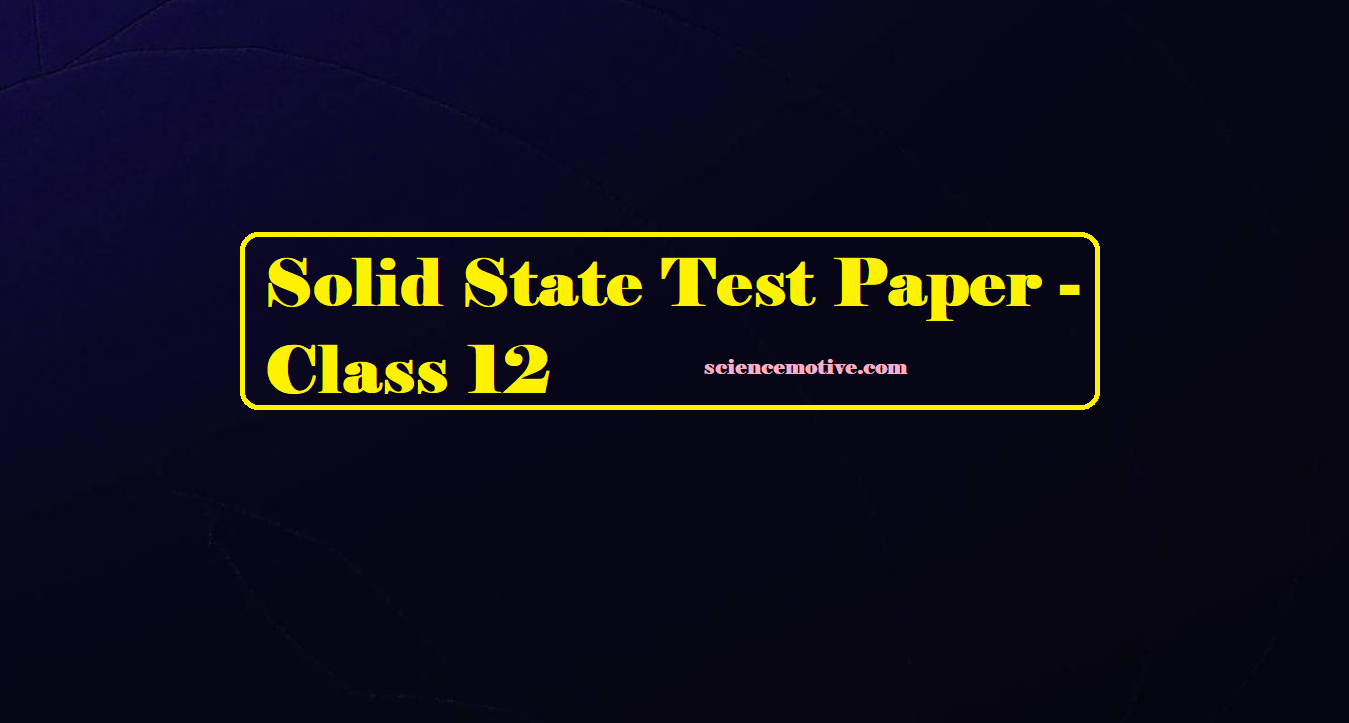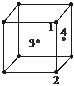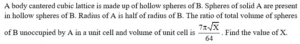# Solid State Test Paper – Class 12# Solid State Test Paper

Solid State Test Paper

Que 1. Solid CO2 is an example of the crystal type –
(A) Ionic                             (B) Covalent
(C) Metallic                        (D) Molecular

Que 2. In an f.c.c. unit cell, atoms are numbered as shown below. The atoms not touching each other are (Atom numbered 3 is face centre of the front face).(A) 3 & 4                                (B) 1 & 3
(C) 1 & 2                                (D) 2 & 4

Que 3. A Schottky defect may involve all but one of the following. Which one is incorrect?
(A) A vacant cation and a vacant anion in an MX lattice.
(B) Vacant cation: anion sites in a ratio of 1:2 in an MX2 lattice.
(C) A vacant cation and vacant anion sites in a ratio of 2:1 in an M2X lattice.
(D) Random vacancies in any lattice.

Que 4. Lithium borohydride (LiBH4), crystallises in an orthorhombic system with 4 molecules per unit cell. The unit cell dimensions are: a = 6.81 Å, b = 4.43 Å, c = 717 Å. If the molar mass of LiBH4 is 21.76 g mol–1. The density of the crystal is-
(A) 0.668 g cm–3
(B) 0.585 g cm2
(C) 1.23 g cm–3
(D) None of these

Que 5. Which statement is incorrect?
(A) FeO is always O deficient.
(B) UO2 tends to have an anion-excess lattice.
(C) TiO is non-stoichiometric.
(D) In a metal deficient M2+O2– lattice, some metal sites contain M3+ ions.

Que 6. If a crystal contains a total of N atoms, and n Schottky defects are produced by removing n cations and r anions from the interior of the crystal, then
(A) n = N exp (-Ep/2kBT)
(B) N = n exp (-Ep/2kBT)
(C) n = N exp (-Ep/kBT)
(D) n = N exp (Ep/kBT)

Solid State Test Paper

Que 7. The number of atoms per unit cell in a simple face centred and body centred cubes is:
(A)  1, 4, 2
(B)  1, 2, 4
(C)  8, 4, 2
(D)  4, 1, 2

Que 8. A metal crystallises with a bcc structure with a density of 19.3 g.cm–3 Calculate the length of the body diagonal of the unit cell. (Atomic weight of metal = 183.9)
(A) 3.21 Å
(B) 4.34 Å
(C) 8.78 Å
(D) 5.48 Å

Que 9. The edge length of the cubic unit cell of lead is 4.92 Å. The density of lead is 11.55 g/cc. What is the type of cubic lattice taken up by lead? (Atomic mass of lead = 207.2.)
(A) fcc
(B) bcc
(C) hcp
(D) bcp

Que 10. Select the correct statement(s) –
(a) The C.N. of cation occupying a tetrahedral hole is 4
(b) The C.N. of cation occupying an octahedral hole is 6.
(c) In Schottky defects, the density of the lattice decreases,
(A) a, b                        (B) b, c
(C) a, b, c                     (D) a, c

Que 11. Sodium metal crystallizes in bcc lattice with cell edge = 4.29Å. The radius of the sodium atom will be-
(A) 1.50 Å
(B) 1.86 Å
(C) 2.80 Å
(D) None of these

Que 12. About half of all the crystals studied so far belong to the ________ crystal system.
(A) hexagonal
(B) orthorhombic
(C) cubic
(D) monoclinic

Que 13. The spinel structure (Ab2O4) consists of an fcc array of O2- ion in which the
(A) A cation occupies one-eighth of the tetrahedral holes and the B cations are the octahedral holes.
(B) A cation occupies one-fourth of the tetrahedral holes and the B cations are the octahedral holes.
(C) A cation occupies one-eighth of the octahedral holes and the B cations are the tetrahedral holes.
(D) A cation occupies one-fourth of the octahedral holes and the B cations are the tetrahedral holes.

Que 14. Choose the correct statement –
(A) Equivalent points in unit cells of a periodic lattice lie on a Bravais lattice.
(B) Equivalent points in unit cells of a periodic lattice do not lie on a Bravais lattice.
(C) There are four Bravais lattices in two dimensions.
(D) There are five Bravais lattices in three dimensions.

Solid State Test Paper

Que 15. A metal crystallizes in two cubic phases, face centred cubic (fcc) and body centred cubic (bcc) whose unit cell lengths are 3.5 and 3.0 Å respectively. Calculate the ratio of the density of fcc and bcc.
(A) 2.123
(B) 1.259
(C) 5.124
(D) 3.134

Que 16. Compute the percentage void space per unit volume of the unit cell in the zinc fluoride structure.
(A) 15.03%
(B) 22.18%
(C) 18.23%
(D) 25.07%

Que 17. Which one is amorphous –
(A) Fe metal
(B) Fused quartz
(C) Wurtzite
(D) NiAs

Que 18. Structure of a compound of the formula A2B  in which each B2– ion is co-ordinated by eight A+ ions and each A+ ion by four B ions  is called
(A) diamagnetic structure
(B) anti-fluorite structure
(C) ferromagnetic structure
(D) paramagnetic structure

Que 19. Consider a body-centred cubic (BCC) arrangement, let de, dfd, dbd be the distances between successive atoms located along the edge, the face-diagonal, and the body diagonal respectively in a unit cell. Their order is given by –
(A) de < dfd < dbd
(B) dfd > dbd > de
(C) dfd > de > dbd
(D) dbd > de > dfd

Que 20. A crystal in which dipoles align themselves in an ordered way so as to give some net dipole moment, on applying a field the direction of polarization changes. Such crystals are called
(A)  Ferroelectric crystals
(B)  Ferroelectric crystals
(C)  Antiferrielectric crystals
(D)  Piezoelectric crystals

For Que 21 – Que 25:
The answer to each question is a NUMERICAL VALUE.

Que 21.  In a face-centred cubic lattice, each lattice point located in a face of the unit cell is shared equally with ________ other unit cells.

Que 22.  If in diamond, there is a unit cell of carbon atoms as fcc and if the carbon atom is sp3, what will be the number of carbon atoms per unit cell?

Que 23.  Silver metal crystallises in a cubic closed packing arrangement with the edge of the unit cell a = 407 pm.  The radius of a silver atom is (X + 3.9) pm. Find the value of X.

Que 24.Que 25.  A crystal formula AB3 has A ions at the cube corners and B ions at the edge centres. The coordination numbers of A and B are respectively 6 and X. Find the value of X.

For Que 26 – Que 40:
State True and False

Que 26. A triclinic crystal system is the most unsymmetrical crystal system.

Que 27. The contribution of an atom at the edge centre in the unit cell is 14.

Que 28. Square close packing in two dimensions is more efficient than the hexagonal close-packed system in two dimensions.

Que 29. The number of octahedral voids in a crystal system is half of the number of tetrahedral voids.

Que 30. SCC has only 26% vacant space.

Que 31. F-centres are responsible for the colour of metal halides.

Que 32. The metal excess defect is a stoichiometric crystal defect.

Que 33. Glass is called pseudo solid.

Que 34. Water is an ionic solid.

Que 35. SiC (Silicon Carbide) is a network solid.

Que 36. Metallic & Ionic crystals both have electrostatic forces of attraction.

Que 37. Vacancy defect results in a decrease in the density of the substance.

Que 38. In Fluorite structure 50% of the tetrahedral voids are occupied by anions.

Que 39. In a 3-dimensional hexagonal close-packed structure all the triangular voids are not covered by the spheres of the second layer.

Que 40. In a 3-dimensional hexagonal close-packed  structure tetrahedral voids are formed when the triangular voids in the second layer lie above the triangular voids in the first layer and the The triangular shapes of these voids do not overlap.

Solid State Test Paper